Next: Example 6.2: Dielectric filled Up: Capacitance Previous: Worked Examples

## Example 6.1: Parallel plate capacitor

Question: A parallel plate capacitor consists of two metal plates, each of area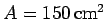, separated by a vacuum gap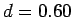cm thick. What is the capacitance of this device? What potential difference must be applied between the plates if the capacitor is to hold a charge of magnitude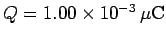on each plate?

Solution: Making use of formula (108), the capacitance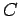is given by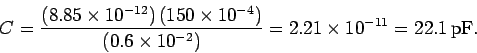The voltage differencebetween the plates and the magnitude of the chargestored on each plate are related via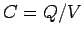, or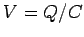. Hence, ifthen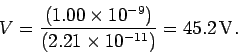Richard Fitzpatrick 2007-07-14